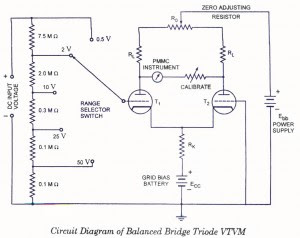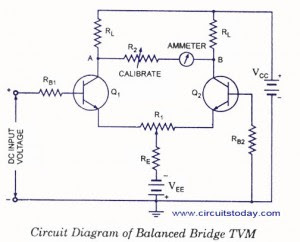## Audio

[Audio][slideshow]

### Balanced Bridge Voltmeters

Such voltmeters can be used for measurement of both dc and ac voltages and are very popular. These voltmeters are available in both versions, vacuum tube type and transistorized type and are described below:

### (a) Balanced Bridge Triode VTVM.

The circuit diagram of a balanced bridge triode VTVM is shown in figure.Balanced Bridge Triode VTVM

In this instrument two identical triodes T1 and T2 are used. Plate voltage is applied to both of the triodes through two, identical resistors RL and a variable resistance RQ. Resistance R0 is so adjusted that plate voltages of both of the triodes become equal when there is no voltage applied on the grid of triode T1. So, in this condition, meter connec­ted between the plates of the two triodes reads zero. The bias battery ECC biases the cathodes of both of the triodes positive and so the bias of the grid of both of the triodes becomes negative with respect to their respective cathodes.

When a positive dc voltage is applied to the grid of triode T1, potential of grid with respect to cathode increases and so the plate current increases. Due to increase in plate current of triode T1 the voltage drop across the resistor RL connected in series with triode T1 increases and, therefore, plate voltage of triode T1 falls. The increased current also flows through the resistor Rk which is common to both of the tubes T1 and T2. An increase in current through resistor Rk causes increase in voltage drop across it and, therefore, cathode of triode T2 becomes more positive. It results in increase of -negative grid bias of tube T2 and therefore, plate current of triode T2 is reduced. With this voltage drop across resistor RL connected with plate of triode T2 falls and, therefore, plate voltage of triode T2 increases. Thus with the application of dc voltage on the grid of triode T1 voltages of the two triode plates do not remain same and a current flows through the PMMC meter, which is proportional to the applied dc voltage. So PMMC meter can be directly calibrated to read the applied input dc voltage. Range of this instrument can be extended by using a range selector switch and a potential divider network. For measuring ac voltage with this voltmeter, it is first rectified and then applied to this voltmeter. A resistance is connected in series with the meter for the purpose of setting the full scale reading of the meter accurately.

The balanced bridge VTVM has the following advantages over an ordinary VTVM (i.e. a VTVM using a single tube):

1. Effect of variations in the tube characteristics is reduced.
2. Fluctuations in the power supply have a smaller effect on the measuring circuit.
3. There is a lesser tendency for the meter zero to shift during the meter operation.

### (b) Balanced Bridge TVM.

The circuit diagram for a balanced bridge TVM is shown in figure.Balanced Bridge TVM

In balanced bridge TVM two identical transistors Q1 and Q2 are used. When the positive input dc voltage is applied to the base of transistor Q1, emitter current of this transistor increases which causes the increase in voltage drop across resistor RL and therefore, reduces the potential of collector of transistor Q1With the increase in emitter current, voltage drop across resistance RE increases and so the emitter current of transistor Q2 is reduced. This increases the potential of the collector of transistor Q2 and a current proportional to the applied input voltage starts flowing in the ammeter connected between collectors of transistors Q1 and Q2.

Resistor R1 is used to achieve zero position in ammeter under the absence of dc input voltage. Resistor R2 is used for calibration purpose.

Main advantage of VTM is that if both transistors Q1 and Q2 are similar then with the change in ambient temperature, p values of both the transistors will change equally so there willbe no effect on collector current in changed ambient temperature condition. Also with the change in ambient temperature, saturation current of both the transistors will change equally and therefore the meter reading will remain unaffected.

Resistor RE also provides negative feedback for transistors Q1 and Q2 as any increase in ambient temperature also increases the emitter current which in turn is limited by the increase in the voltage drop across resistor RE which is normally of high magnitude. Thus accuracy of calibration of TVM is maintained over a wide range of temperature.

Another advantage of TVM is that any fluctuation in power supply VCC and VEE does not affect its performance as it changes the biasing of both the transistors equally. Main disadvantage of balanced bridge TVM is that it offers less input impedance comparatively. But by using FET in the circuit of TVM, very high input impedance can be achieved. In the circuit of this TVM, two resistors RL and the internal resistances of transistors Q1 and Q2 form the arms of a balanced bridge and the current flows through the meter when external voltage is applied to the base of transistor Q1, which disturbs the balance of the bridge. That is why, this is called the balanced bridge TVM.

[Lights][grids]